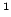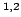Statistica Sinica 12(2002), 965-990

UNIMODAL DENSITY ESTIMATION

USING KERNEL METHODS

Peter Halland Li-Shan HuangAustralian National University andUniversity of Rochester

Abstract: We suggest a method for rendering a standard kernel density estimator unimodal: tilting the empirical distribution. It is proposed that the amount of tilting be chosen in order to minimise, subject to unimodality, the integrated squared distance between a conventional density estimator and its tilted version. This approach has an interesting data-compression aspect, in that the algorithm often implicitly summarises the dataset in a relatively small subsample as part of the process of enforcing unimodality. Another feature is that, no matter what the chosen bandwidth, the algorithm produces (with probability 1, for each sample size) a proper density estimate. Thus, it may be employed as an adjunct to any of the many popular bandwidth selection rules for density estimation. We show theoretically that in classes of densities that are of practical interest, the method enhances performance without suffering any deleterious first-order impact on asymptotic accuracy, for example as reflected in integrated squared error. In such cases, and except in places where the true density is virtually flat, the constrained density estimate is first-order equivalent to its unconstrained counterpart. The case where the number of modes is constrained to equal a number greater than 1 is also considered.

Key words and phrases: Bandwidth, biased bootstrap, integrated squared error, mode, nonparametric density estimation, order restricted inference, power divergence, tilting, weighted bootstrap.